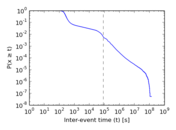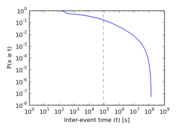# Last.fm bands

This bipartite network contains user–band listening events from the music website last.fm. An edge shows that a user listened to a song of a band.

 Code `Lb` Internal name `lastfm_band` Name Last.fm bands Data source http://www.dtic.upf.edu/~ocelma/MusicRecommendationDataset/lastfm-1K.html AvailabilityDataset is available for download Consistency checkDataset passed all tests Category Interaction network Node meaning User, band Edge meaning Listening Network formatBipartite, undirected Edge typeUnweighted, multiple edges Temporal dataEdges are annotated with timestamps

## Statistics

 Size n = 175,069 Left size n1 = 992 Right size n2 = 174,077 Volume m = 19,150,868 Unique edge count m̿ = 898,062 Wedge count s = 889,895,853 Claw count z = 890,736,635,531 Cross count x = 976,152,225,850,783 Square count q = 4,419,565,780 4-Tour count T4 = 38,918,474,416 Maximum degree dmax = 183,103 Maximum left degree d1max = 183,103 Maximum right degree d2max = 115,209 Average degree d = 218.781 Average left degree d1 = 19,305.3 Average right degree d2 = 110.014 Fill p = 0.005 200 60 Average edge multiplicity m̃ = 21.324 7 Size of LCC N = 175,069 Diameter δ = 6 50-Percentile effective diameter δ0.5 = 3.475 46 90-Percentile effective diameter δ0.9 = 3.895 35 Median distance δM = 4 Mean distance δm = 3.919 94 Gini coefficient G = 0.964 193 Balanced inequality ratio P = 0.060 768 4 Left balanced inequality ratio P1 = 0.289 593 Right balanced inequality ratio P2 = 0.089 095 2 Relative edge distribution entropy Her = 0.760 117 Power law exponent γ = 2.581 27 Tail power law exponent γt = 1.811 00 Tail power law exponent with p γ3 = 1.811 00 p-value p = 0.000 00 Left tail power law exponent with p γ3,1 = 1.421 00 Left p-value p1 = 0.000 00 Right tail power law exponent with p γ3,2 = 1.881 00 Right p-value p2 = 0.000 00 Degree assortativity ρ = −0.177 727 Degree assortativity p-value pρ = 0.000 00 Spectral norm α = 27,811.3 Algebraic connectivity a = 0.878 651 Spectral separation |λ1[A] / λ2[A]| = 1.003 32 Controllability C = 173,085 Relative controllability Cr = 0.988 667

## Plots

### Fruchterman–Reingold graph drawing### Degree distribution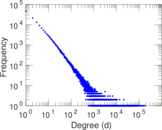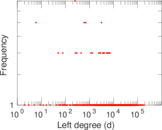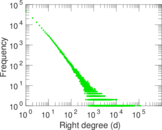### Cumulative degree distribution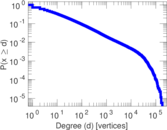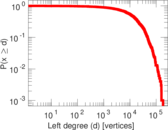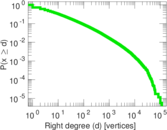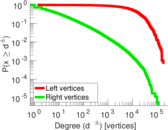### Lorenz curve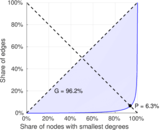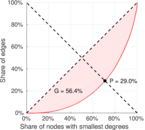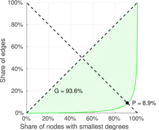### Spectral distribution of the adjacency matrix### Spectral distribution of the normalized adjacency matrix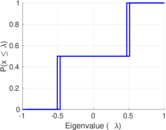### Spectral distribution of the Laplacian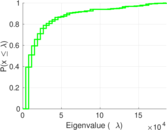### Spectral graph drawing based on the adjacency matrix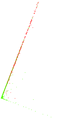### Spectral graph drawing based on the Laplacian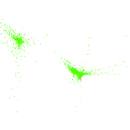### Spectral graph drawing based on the normalized adjacency matrix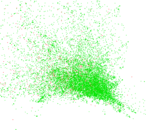### Degree assortativity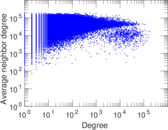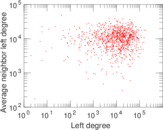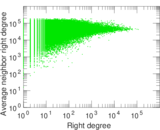### Zipf plot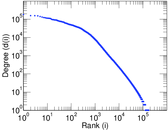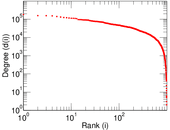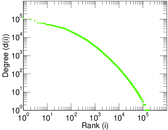### Hop distribution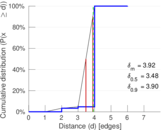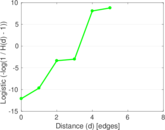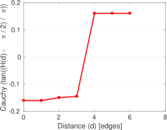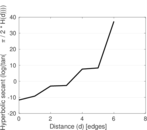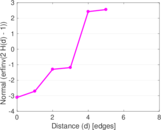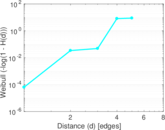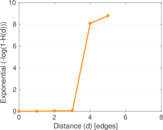### Edge weight/multiplicity distribution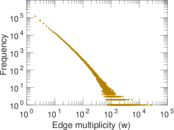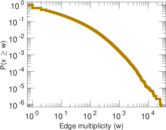### Temporal distribution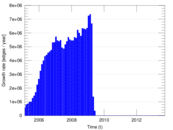### Temporal hop distribution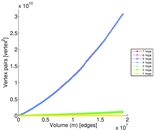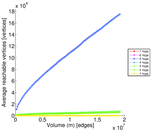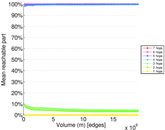### Diameter/density evolution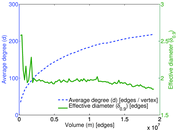### Inter-event distribution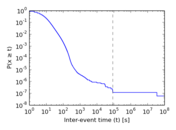### Node-level inter-event distribution